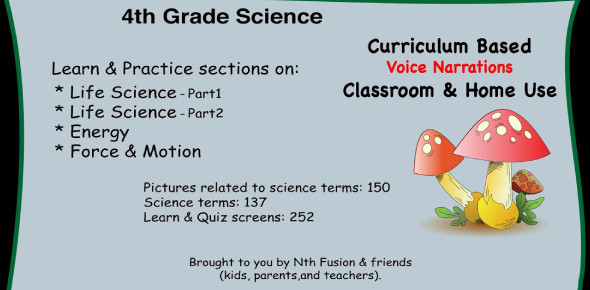# 4th Grade Science Test On Electricity! Trivia Quiz

19 Questions | Attempts: 5198SettingsElectricity is something that all age groups should learn. Regarding this quiz, you will have to discern a parallel unit, a series, an electric diagram versus a circuit diagram, what is a good insulator, what is a good conductor, and what is a material through which electric current cannot easily pass. This quiz will help you turn on the light and see what electricity is all about.

• 1.
Electric current in a/an ____________________ circuit travels in MORE THAN ONE path.
• A.

Series

• B.

Wire

• C.

Parallel

• D.

Connected

• 2.
In a/an ________________________ circuit, if one bulb burns out, the others will stay lit.
• A.

Parallel

• B.

Series

• 3.
In a _________________________ circuit, if one bulb burns out, the others will not work.
• A.

Parallel

• B.

Series

• 4.
A drawing that uses symbols to show how the parts of an electric circuit are connected is a/an _____________________________.
• A.

Nichrome wire

• B.

Electric diagram

• C.

Parallel circuit

• D.

Circuit diagram

• 5.
Which of the following is NOT a good insulator?
• A.

Plastic

• B.

Glass

• C.

Iron

• 6.
Which of the following is NOT a good conductor?
• A.

Iron

• B.

Copper

• C.

Plastic

• 7.
Electric current in a/an _____________________ circuit travels in ONE path.
• A.

Series

• B.

Parallel

• C.

Electric

• D.

Wire

• 8.
A device used to indicate a closed path across two points in a circuit is a/an ______________________.
• A.

Circuit diagram

• B.

Filament

• C.

Nichrome wire

• D.

Circuit tester

• 9.
A material through which electric current CANNOT pass easily is a/an ____________________.
• A.

Tester

• B.

Insulator

• C.

Receptor

• D.

Conductor

• 10.
A material through which electric current CAN pass easily in a/an ______________________
• A.

Tester

• B.

Insulator

• C.

Receptor

• D.

Conductor

• 11.
___________________________ wire is made of nickel and chromium.
• A.

Copperchrome

• B.

Nichrome

• C.

Cochrome

• D.

Nikelchrome

• 12.
A fine wire that becomes hot and produces light when an electric current passes through it is called a _________________.
• A.

Filament

• B.

Nichrome wire

• C.

Circuit

• D.

Wire

• 13.
In order for a circuit to work, the path must be __________________.
• A.

Series

• B.

Paralled

• C.

Open

• D.

Closed

• 14.
A/An _____________________ is used to turn the electricity in a current on or off.
• A.

Battery

• B.

Switch

• C.

Conductor

• D.

Insulator

• 15.
The flow of electric charge through something is a/an __________________________.
• A.

Electric current

• B.

Circuit tester

• C.

Battery

• D.

Wire

• 16.
A metal strand used to connect electrical devices in a circuit is a/an ________________________.
• A.

Battery

• B.

Switch

• C.

Filament

• D.

Wire

• 17.
A power source than can produce an elecctric current as a result of a chemical change in it is a/an ______________________.
• A.

Circuit

• B.

Wire

• C.

Battery

• D.

Filament

• 18.
The path for electricity to travel along is a/an ________________________.
• A.

Electric current

• B.

Circuit

• C.

Closed circuit

• D.

Electric diagram

• 19.
A circuit must have which of the following?
• A.

Energy source, battery, something to work

• B.

Energy source, wire, circuit tester

• C.

Energy source, wire, switch

• D.

Energy source, wire, something to work

## Related TopicsBack to top
×

Wait!
Here's an interesting quiz for you.